Home Page Introduction Classical Ciphers Enigma Machine DES Key Agreement PGP R.S.A. Number Theory Other Links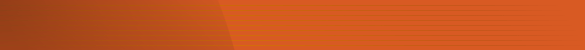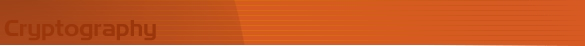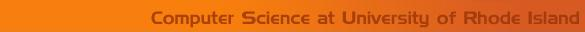Simplified DES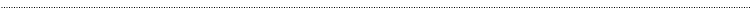Introduction

Simplified DES is an algorithm explained in Section 4.2 of , is an algorithm that has many features of the DES, but is much simpler then DES. Like DES, this algorithm is also a bock cipher.

Block Size:  In Simplified DES, encryption/decryption is done on blocks of 12 bits. The plaintext/ciphertext is divided into blocks of 12 bits and the algorithm is applied to each block.

Key: - The key has 9 bits. The key, Ki, for the ith round of encryption is obtained by using 8 bits of K, starting with the ith bit.

Example: If K = 111000111

Then K1 = 11100011 and K3 = 10001111 and K10 = K1 = 11100011

Algorithm

The block of 12 bits is written in the form  L0R0, where L0 consists of the first 6 bits and R0 consists of the last 6 bits. The ith round of the algorithm transforms an input Li-1Ri-1 to the output LiRi using an 8-bit Ki derived from K.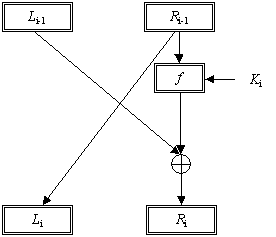One Round of a Feistel System

The output for the ith round is found as follows.

Li = Ri-1 and Ri = Li-1 Å f (Ri-1, Ki)

This operation is performed for a certain number of rounds, say n, and produces LnRn. The ciphertext will be RnLn. Encryption and decryption are done the same way except the keys are selected in the reverse order. The keys for encryption will be K1, K2  Kn and for decryption will be Kn, Kn-1  K1.

Function f(Ri-1,Ki): - The function f(Ri-1,Ki), depicted in the Figure below, is described in following steps.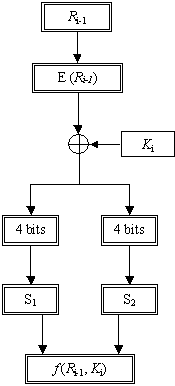The Function f (Ri-1, Ki)

1. The 6-bits are expanded using the following expansion function. The expansion function takes 6-bit input and produces an 8-bit output. This output is the input for the two S-boxes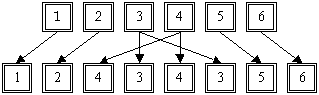The Expansion Function, E(Ri-1)

1. The 8-bit output from the previous step is Exclusive-ORed with the key Ki

2. The 8-bit output is divided into two blocks. The first block consists of the first 4 bits and the last four bits make the second block. The first block is the input for the first S-box (S1) and the second block is the input for the second S-box (S2).

3. The S-boxes take 4 bits as input and produce 3bits of output.  The first bit of the input is used to select the row from the S-box, 0 for the first row and 1 for the second row. The last 3 bits are used to select the column.

Example: Let the output from the expander function be 11010010.

So  1101 will be the input for the S1 box and 0010 will be the input for the S2 box.  The output from the S1 box will be 111, the first of the input is 1 so select the second  row and 101 will select the 6th column. Similarly the output from the S2 box will be 110.

1. The output from the S-boxes is combined to form a single block of 6 bits. These 6 bits will be the output of the function f(Ri-1,Ki).

In our example we have the S1 output 111 and S2 output 110. So the output for the function f(Ri-1,Ki) will be 111110, the S1 output followed by the S2 output.

The S1 and S2 boxes are shown below.

S1-Box

 101 010 001 110 011 100 111 000 001 100 110 010 000 111 101 011

S2-Box

 100 000 110 101 111 001 011 010 101 011 000 111 110 010 001 100

Example

The example is explained for two rounds.

Let Input message be 100010110101 and the key be 111000111.

Encryption

Round 1  (i = 0)

1. L0= 100010 and R0= 110101; K1 = 11100011.

2. E(R0) = 11101001.

3. E(R0)  Å  K1  = 11101001 Å 11100011 = 00001010.

4. S1(0000) = 101, S2(1010) = 000, è f(R0,K1) = 101000

5. f(R0,K1) Å L0 = 101000 Å 100010 = 001010.

6. Now using the formulas Li = Ri-1 and Ri = Li-1 Å f (Ri-1, Ki)

we get L1 = 110101 and R1 = 001010.

Round 2  (i = 1)

1. L1 = 110101 and R1 = 001010; K2 = 11000111.

2. E(R1) = 00010110.

3. E(R1)  Å  K1  = 00010110 Å 11000111= 11010001.

4. S1(1101) = 111; S2(0001) = 000; è f(R1,K2) = 111000

5. f(R1,K2) Å L1 = 111000 Å 110101 = 001101.

6. Now using the formulas Li = Ri-1 and Ri = Li-1 Å f (Ri-1, Ki)

we get L2 = 001010 and R2 = 001101.

So encrypted message, R2 L2 = 001101001010

Decryption

Round 1(i = 0)

1. L0= 001101 and R0= 001010; K2 = 11000111.

2. E(R0) = 00010110.

3. E(R0)  Å  K1  = 00010110 Å 11000101 = 11010001.

4. S1(1101) = 121; S2(0001) = 000; è f(R0,K2) = 111000

5. f(R0,K2) Å L0 = 111000 Å 001101 = 110101.

6. Now using the formulas Li = Ri-1 and Ri = Li-1 Å f (Ri-1, Kn-i)

we get L1 = 001010 and R1 = 111000.

Round 2 (i = 1)

1. L1 = 001010 and R1 = 111000; K1 = 11100011.

2. E(R1) = 00010110.

3. E(R1)  Å  K1  = 11101001 Å 11100011= 00001010.

4. S1(0000) = 101; S2(1010) = 000; è f(R1,K1) = 101000

5. f(R1,K1) Å L1 = 101000 Å 001010 = 100010.

6. Now using the formulas Li = Ri-1 and Ri = Li-1 Å f (Ri-1, Kn-i)

we get L2 = 110101 and R2 = 100010.

So decrypted message, R2 L2 =100010110101, which is the original plaintext message.